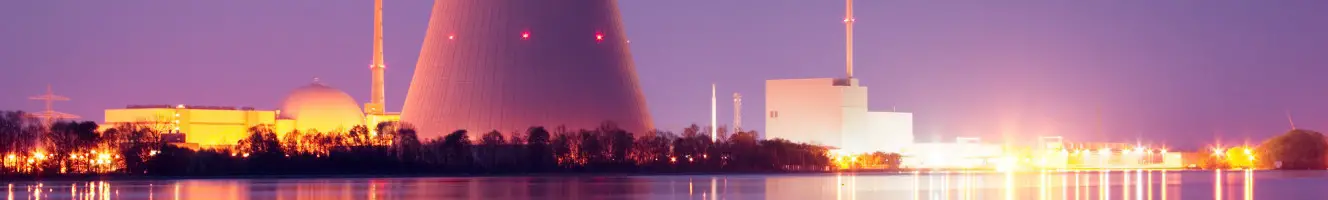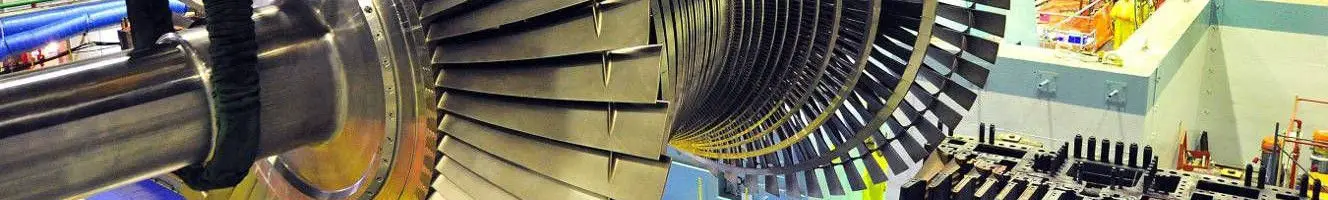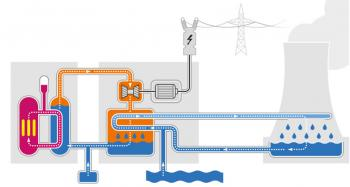# Nuclear EnergyNuclear Power Plant Isar, GermanySpent nuclear fuel poolTurbine of a nuclear plant

# Kinetic

## Kinetic energyKinetic energy is the energy contained in a body due to being in motion. The kinetic energy is the amount of work needed to accelerate a body of a given mass to a certain speed (linear or rotational). This energy obtained during acceleration will remain unchanged as long as this body does not change its speed.

In classical mechanics, the linear kinetic energy (without rotation) of an object of mass m traveling at velocity v is ½ · m · v2. In relativistic mechanics it is a good approximation only when the speed is much less than the speed of light.

+ info

## Mechanical energyMechanical energy can be defined as the ability to produce a mechanical work that a body possesses due to causes of mechanical origin, such as its position or its speed. There are two forms of mechanical energy that are kinetic energy and potential energy.

The mechanical energy of a body is the sum of its kinetic energy and its potential energy. Mechanical energy can be partially transformed into other types of energy, such as electrical energy (in a ventilator), and can be obtained by transforming other energies, such as chemical energy (in a person who walks).

The kinetic energy…

+ info

## Definition of energyAbility of a physical system to produce a job.

This definition, although it is the most widespread, can be misleading because of the vagueness of the term "capacity", and therefore, it is better to define the energy as what, when a work occurs, decreases in an amount equal to the work produced. Energy, then, is measured in the same units as labor.

History of the study of energy

The notion of energy appears for the first time from the investigations of Joule and Carnot on the conversion of heat in mechanical work and thanks to the theory of Helmholtz in which it connects…

+ info

## Potential energyIn physics, the potential energy is the energy that an object possesses due to its position in a force field or that a system has due to the configuration of its parts.

There are many types of potential energy, but the most common are:

• Gravitational potential energy that depends on the vertical position and the mass of an object.
• Elastic potential energy of an extended spring
• Electric potential energy of a charge in an electric field.

The unit of measurement of the International System of Units for energy is the joule (J).

Potential…

+ info

## Neutron moderatorThe moderator is a component that is part of nuclear reactors. It is located in the reactor core. The function of the moderator is to reduce the speed of neutrons in nuclear fission reactions.

During fission nuclear reactions, neutrons collide with fissile atoms (uranium and plutonium) present in the nuclear fuel causing fission. With each fission reaction, one or two neutrons are released at high speed.

The objective to maintain a chain fission reaction is that these neutrons hit other fissile atoms but at such a speed it is very difficult. The objective of…

+ info

## ThermodynamicsThermodynamics is the branch of classical physics that studies and describes the thermodynamic transformations induced by heat and work in a thermodynamic system, as a result of processes that involve changes in the state variables of temperature and energy.

Classical thermodynamics is based on the concept of macroscopic system, that is, a portion of physical mass or conceptually separated from the external environment, which is often assumed for convenience that is not disturbed by the exchange of energy with the system. The state of a macroscopic system that is in equilibrium conditions…

+ info

## Nuclear Power Plant WorkingNowadays, the main use given to nuclear energy is the generation of electric power. Nuclear power plants are responsible of doing this process. Almost all nuclear power plants in production are using nuclear fission since the nuclear fusion, despite being under development, is currently unfeasible.

The operation of a nuclear power plant is identical to the operation of a thermoelectric power plant working with fossil fuels like coal, oil or gas, except in the way of providing heat to the water for converting this one into steam. In nuclear reactors this process of producing heat is made…

+ info